# Notebook 17: Energy-based Generative Models for MNIST¶

## Learning Goal¶

The goal of this notebook is to familiarize readers with various energy-based generative models including: Restricted Boltzmann Machines (RBMs) with Gaussian and Bernoulli units, Deep Boltzmann Machines (DBMs), as well as techniques for training these model including contrastive divergence (CD) and persistent constrastive divergence (PCD). We will also discuss how to generate new examples (commonly called fantasy particles in the ML literature). The notebook also introduces the Paysage package from UnLearn.AI for quickly building and training these models..

## Overview¶

In this notebook, we study the MNIST dataset using generative models.

Let us adopt a different perspective on the MNIST dataset. Generative models, as the name suggests, are useful to generate brand new data (images) by learning how to imitate the ones from a given data set. If you wish, they can be regarded as a kind of "creative ML". This is achieved by extracting/learning a set of features which represent the backbone of the entire data and are thus definitive for the particular dataset. These features are encoded in the weights of the model.

Mathematically speaking, generative models are designed and trained to learn an approximation for the probability distribution that generated the data. Intuitively, this task is much more complex and sophisticated than the image recognition task. Therefore, we shall approach the problem in a number of steps of increasing complexity. For a more detailed discussion on the theory, we invite the reader to check out Secs. XV and XVI of the review.

Below, we analyze four different generative models: the Hopfield Model, a Restricted Boltzmann Machine (RBM), a regularized RMB with sparse weights, and a Deep Boltzmann Machine (DBM). In all these cases, we first set up and train the models. After that, we compare the results. Last, we open up the black box of each model and visualise a set of features it learned.

## Setting up Paysage¶

In this notebook, we use an open-source python package for energy-based models, called paysage. Paysage requires python>3.5; we recommend using the package with an Anaconda environment.

To install paysage:

• activate an Anaconda3 environment
• navigate to the directory which contains the paysage files
• and execute
pip install .

Documentation for paysage is available under https://github.com/drckf/paysage/tree/master/docs.

By default, computations in paysage are performed using numpy/numexpr/numba on the CPU. If you have installed PyTorch, then you can switch to the pytorch backend by changing the setting in paysage/backends/config.json to pytorch.

Let us set up the required packages for this notebook by importing paysage.

In :
from __future__ import print_function, division
import os
import paysage
import numpy as np
import pandas as pd

# for Boltzmann machines
from paysage import preprocess as pre
from paysage.layers import BernoulliLayer, GaussianLayer
from paysage.models import BoltzmannMachine
from paysage import batch
from paysage import fit
from paysage import optimizers
from paysage import samplers
from paysage import backends as be
from paysage import schedules
from paysage import penalties as pen

# fix random seed to ensure deterministic behavior
np.random.seed(137)
be.set_seed(137)


## Obtaining the MNIST dataset¶

As we mentioned in the introduction, we use the MNIST dataset of handwritten digits to study the Hopfield model and various variants of RBMs.

The MNIST dataset comprises $70000$ handwritten digits, each of which comes in a square image, divided into a $28\times 28$ pixel grid. Every pixel can take on $256$ nuances of the grey colour, interpolating between white and black, and hence each data point assumes any value in the set $\{0,1,\dots,255\}$. There are $10$ categories in the problem, corresponding to the ten digits. In previous notebooks, we formulated a classification task for the MNIST dataset, and studied it using discriminative supervised learning: Logistic Regression and Deep Neural Networks.

This dataset can be fetched using paysage from the web as an HDF5 file. For this, you should

• navigate further into the /examples/mnist/ directory which contains the file download_mnist.py.
• from the terminal, run the command
python3 /examples/mnist/download_mnist.py

This file contains keys train/images, train/labels, test/images, and test/labels and is compressed to about 15 Mb in size.

As our first step, we will set up the paths to the data and shuffle the dataset. The shuffled dataset will not be compressed (for faster reading during training) so it will be about 56 Mb in size.

Why shuffle the data? - Training with stochastic gradient descent means we will be using small minibatches of data (maybe 50 examples) to compute the gradient at each step. If the data have an order, then the estimates for the gradients computed from the minibatches will be biased. Shuffling the data ensures that the gradient estimates are unbiased (though still noisy).

In :
paysage_path = os.path.dirname(os.path.dirname(paysage.__file__))
mnist_path = os.path.join(paysage_path, "examples", "mnist", "mnist.h5")
shuffled_mnist_path = os.path.join(paysage_path, "examples", "mnist", "shuffled_mnist.h5")

print("path to mnist data:")
print(mnist_path)

if not os.path.exists(mnist_path):
raise IOError("{} does not exist. run mnist/download_mnist.py to fetch from the web".format(mnist_path))

if not os.path.exists(shuffled_mnist_path):
batch.DataShuffler(mnist_path, shuffled_mnist_path, complevel=0).shuffle()

path to mnist data:


### Processing the Data¶

Next, we create a data generator, which splits the data into a training and validation sets, and separates them into minibatches of size batch_size. Before we begin training, we set data into training mode.

To monitor the progress of performance metrics during training, we define the variable performance which tells Paysage to measure the reconstruction error from the validation set. Possible metrics include the reconstruction error (used in this example) and metrics related to difference in energy of random samples and samples from the model (see metrics.md in Paysage documentation for a complete list).

In :
##### set up minibatch data generator
# batch size
batch_size=100
transform = pre.Transformation(pre.binarize_color)

# create data generator object with minibathces
data = batch.in_memory_batch(samples, batch_size, train_fraction=0.95, transform=transform)

# reset the data generator in training mode
data.reset_generator(mode='train')

/anaconda3/lib/python3.6/site-packages/ipykernel_launcher.py:7: FutureWarning: Method .as_matrix will be removed in a future version. Use .values instead.
import sys


### Setting up a Hopfield Model¶

Having loaded and preprocessed the data, we now move on to construct a hopfield model. To do this, we use the Model class and with a visible BernoulliLayer and a hidden GaussianLayer. Note that the visible layer has the same size as the input data points, which is can be read off data.ncols. The number of hidden units is num_hidden_units. We also set the mean and variance of the Gaussian layer to zero and unity, respectively (the notation here is inspired by the terminology in Variational Auto Encoders).

We choose to train the model with the Adam optimizer. To ensure convergence, we attenuate the learning_rate hyperparameter according to a PowerLawDecay schedule: learning_rate$(t)$ =initial$/(1 +$ coefficient$\;\times\; t)$. It will prove convenient to define the function Adam_optimizer() for this purpose.

In :
##### create hopfield model
# hidden units
num_hidden_units=200

# set up the model
vis_layer = BernoulliLayer(data.ncols)
hid_layer = GaussianLayer(num_hidden_units)
hopfield = BoltzmannMachine([vis_layer, hid_layer])

# set mean and standard deviation of hidden layer to to 0 and 1, respectively
hopfield.layers.set_fixed_params(['loc', 'log_var'])

# set up an optimizer method (ADAM in this case)
# define learning rate attenuation schedule
learning_rate=schedules.PowerLawDecay(initial=initial,coefficient=coefficient)
# return optimizer object


### Compiling and Training a Model in Paysage¶

Next, we have compile the model. First, we initialize a model using the initialize function attribute which accepts the data as a required argument. We choose the initialization routine glorot, see discussion in review. Second, we define an optimizer calling the function Adam_optimizer() defined above, and store the object under the name opt. To define a Monte Carlo sampler, we use the method from_batch of the SequentialMC class, parsing the model and the data. Last, we create an SGD object called trainer to train the model using Persistent Contrastive Divergence (pcd) with a fixed number of monte_carlo_steps. We can also monitor the reconstruction error during training. Last, we train the model in epochs (see variable nim_epochs), calling the train() method of trainer. These steps are universal for shallow generative model's, and it is convenient to combine them in the function train_model().

In :
# define function to compile and train model
num_epochs=20 # training epochs
monte_carlo_steps=1 # number of MC sampling steps
def train_model(model,num_epochs,monte_carlo_steps):
# make a simple guess for the initial parameters of the model
model.initialize(data,method='glorot_normal')
# set optimizer
trainer = fit.SGD(model, data)
trainer.train(opt, num_epochs, method=fit.pcd, mcsteps=monte_carlo_steps)
# train hopfield model
train_model(hopfield,num_epochs,monte_carlo_steps)

Before training:
-ReconstructionError: 1.203098
-EnergyCoefficient: 0.134485
-HeatCapacity: 0.434479
-WeightSparsity: 0.335712
-WeightSquare: 1.588543
-KLDivergence: 0.188799
-ReverseKLDivergence: -0.036247

End of epoch 1:
Time elapsed 5.769s
-ReconstructionError: 0.758059
-EnergyCoefficient: 0.271930
-HeatCapacity: 8.722163
-WeightSparsity: 0.268762
-WeightSquare: 2.355603
-KLDivergence: 0.089433
-ReverseKLDivergence: 0.153994

End of epoch 2:
Time elapsed 5.785s
-ReconstructionError: 0.717784
-EnergyCoefficient: 0.290698
-HeatCapacity: 12.103565
-WeightSparsity: 0.268668
-WeightSquare: 2.623842
-KLDivergence: 0.077823
-ReverseKLDivergence: 0.192197

End of epoch 3:
Time elapsed 5.529s
-ReconstructionError: 0.720571
-EnergyCoefficient: 0.355613
-HeatCapacity: 9.184643
-WeightSparsity: 0.267966
-WeightSquare: 2.789534
-KLDivergence: 0.071486
-ReverseKLDivergence: 0.328846

End of epoch 4:
Time elapsed 5.623s
-ReconstructionError: 0.721592
-EnergyCoefficient: 0.315879
-HeatCapacity: 19.097582
-WeightSparsity: 0.267072
-WeightSquare: 2.881694
-KLDivergence: 0.089170
-ReverseKLDivergence: 0.226370

End of epoch 5:
Time elapsed 5.985s
-ReconstructionError: 0.731511
-EnergyCoefficient: 0.352591
-HeatCapacity: 12.398974
-WeightSparsity: 0.270751
-WeightSquare: 2.860990
-KLDivergence: 0.101515
-ReverseKLDivergence: 0.312168

End of epoch 6:
Time elapsed 5.66s
-ReconstructionError: 0.766730
-EnergyCoefficient: 0.329614
-HeatCapacity: 12.116742
-WeightSparsity: 0.278617
-WeightSquare: 2.792415
-KLDivergence: 0.058859
-ReverseKLDivergence: 0.252365

End of epoch 7:
Time elapsed 5.785s
-ReconstructionError: 0.722264
-EnergyCoefficient: 0.353819
-HeatCapacity: 8.638835
-WeightSparsity: 0.279320
-WeightSquare: 2.912473
-KLDivergence: 0.062427
-ReverseKLDivergence: 0.300098

End of epoch 8:
Time elapsed 5.471s
-ReconstructionError: 0.736371
-EnergyCoefficient: 0.310364
-HeatCapacity: 154.251839
-WeightSparsity: 0.276663
-WeightSquare: 3.021360
-KLDivergence: 0.082398
-ReverseKLDivergence: 0.249388

End of epoch 9:
Time elapsed 5.657s
-ReconstructionError: 0.715077
-EnergyCoefficient: 0.357051
-HeatCapacity: 45.662843
-WeightSparsity: 0.274935
-WeightSquare: 3.170220
-KLDivergence: 0.072203
-ReverseKLDivergence: 0.402410

End of epoch 10:
Time elapsed 5.924s
-ReconstructionError: 0.701699
-EnergyCoefficient: 0.213532
-HeatCapacity: 44.744286
-WeightSparsity: 0.267844
-WeightSquare: 3.424128
-KLDivergence: 0.042195
-ReverseKLDivergence: 0.176381

End of epoch 11:
Time elapsed 5.874s
-ReconstructionError: 0.704685
-EnergyCoefficient: 0.287322
-HeatCapacity: 49.121368
-WeightSparsity: 0.261188
-WeightSquare: 3.559100
-KLDivergence: 0.039975
-ReverseKLDivergence: 0.235038

End of epoch 12:
Time elapsed 5.414s
-ReconstructionError: 0.704191
-EnergyCoefficient: 0.335872
-HeatCapacity: 70.598681
-WeightSparsity: 0.257006
-WeightSquare: 3.789706
-KLDivergence: 0.086966
-ReverseKLDivergence: 0.332300

End of epoch 13:
Time elapsed 5.503s
-ReconstructionError: 0.691235
-EnergyCoefficient: 0.290370
-HeatCapacity: 21.146751
-WeightSparsity: 0.254605
-WeightSquare: 4.006200
-KLDivergence: 0.028466
-ReverseKLDivergence: 0.208005

End of epoch 14:
Time elapsed 5.538s
-ReconstructionError: 0.691635
-EnergyCoefficient: 0.324749
-HeatCapacity: 57.623025
-WeightSparsity: 0.254884
-WeightSquare: 4.318763
-KLDivergence: 0.024234
-ReverseKLDivergence: 0.316771

End of epoch 15:
Time elapsed 6.203s
-ReconstructionError: 0.688565
-EnergyCoefficient: 0.370633
-HeatCapacity: 38.042551
-WeightSparsity: 0.256465
-WeightSquare: 4.470937
-KLDivergence: 0.109225
-ReverseKLDivergence: 0.387763

End of epoch 16:
Time elapsed 5.797s
-ReconstructionError: 0.668726
-EnergyCoefficient: 0.300863
-HeatCapacity: 259.275070
-WeightSparsity: 0.259555
-WeightSquare: 4.877840
-KLDivergence: 0.046502
-ReverseKLDivergence: 0.313053

End of epoch 17:
Time elapsed 6.329s
-ReconstructionError: 0.698139
-EnergyCoefficient: 0.503270
-HeatCapacity: 439.002197
-WeightSparsity: 0.264087
-WeightSquare: 5.104922
-KLDivergence: 0.169157
-ReverseKLDivergence: 0.636722

End of epoch 18:
Time elapsed 6.002s
-ReconstructionError: 0.687114
-EnergyCoefficient: 0.279782
-HeatCapacity: 59.622955
-WeightSparsity: 0.265842
-WeightSquare: 5.337249
-KLDivergence: 0.029801
-ReverseKLDivergence: 0.267772

End of epoch 19:
Time elapsed 9.392s
-ReconstructionError: 0.675484
-EnergyCoefficient: 0.308026
-HeatCapacity: 496.223302
-WeightSparsity: 0.274207
-WeightSquare: 5.625677
-KLDivergence: 0.077853
-ReverseKLDivergence: 0.308562

End of epoch 20:
Time elapsed 5.745s
-ReconstructionError: 0.666614
-EnergyCoefficient: 0.314069
-HeatCapacity: 407.979512
-WeightSparsity: 0.267616
-WeightSquare: 6.095773
-KLDivergence: 0.087877
-ReverseKLDivergence: 0.308343



## More Generative Models¶

We can easily create a Bernoulli RBM and train it using the functions defined above as follows:

In :
##### Bernoulli RBM
vis_layer = BernoulliLayer(data.ncols)
hid_layer = BernoulliLayer(num_hidden_units)
rbm = BoltzmannMachine([vis_layer, hid_layer])

# train Bernoulli RBM
train_model(rbm,num_epochs,monte_carlo_steps)

Before training:
-ReconstructionError: 1.224468
-EnergyCoefficient: 0.130849
-HeatCapacity: 0.310575
-WeightSparsity: 0.335764
-WeightSquare: 1.586147
-KLDivergence: 0.172399
-ReverseKLDivergence: -0.036571

End of epoch 1:
Time elapsed 4.522s
-ReconstructionError: 0.883955
-EnergyCoefficient: 0.332970
-HeatCapacity: 0.419587
-WeightSparsity: 0.228966
-WeightSquare: 27.294800
-KLDivergence: 0.141439
-ReverseKLDivergence: 0.220725

End of epoch 2:
Time elapsed 4.698s
-ReconstructionError: 0.811407
-EnergyCoefficient: 0.321183
-HeatCapacity: 0.880261
-WeightSparsity: 0.228917
-WeightSquare: 48.228872
-KLDivergence: 0.054885
-ReverseKLDivergence: 0.255241

End of epoch 3:
Time elapsed 4.524s
-ReconstructionError: 0.772367
-EnergyCoefficient: 0.358062
-HeatCapacity: 0.704555
-WeightSparsity: 0.227952
-WeightSquare: 68.845010
-KLDivergence: 0.097184
-ReverseKLDivergence: 0.276903

End of epoch 4:
Time elapsed 4.394s
-ReconstructionError: 0.740070
-EnergyCoefficient: 0.308440
-HeatCapacity: 1.553747
-WeightSparsity: 0.222856
-WeightSquare: 92.983086
-KLDivergence: 0.066805
-ReverseKLDivergence: 0.251889

End of epoch 5:
Time elapsed 4.49s
-ReconstructionError: 0.731708
-EnergyCoefficient: 0.320784
-HeatCapacity: 0.859078
-WeightSparsity: 0.219865
-WeightSquare: 119.025088
-KLDivergence: 0.090319
-ReverseKLDivergence: 0.275634

End of epoch 6:
Time elapsed 4.493s
-ReconstructionError: 0.713038
-EnergyCoefficient: 0.305011
-HeatCapacity: 2.768552
-WeightSparsity: 0.217180
-WeightSquare: 142.648643
-KLDivergence: 0.069115
-ReverseKLDivergence: 0.265705

End of epoch 7:
Time elapsed 4.408s
-ReconstructionError: 0.688988
-EnergyCoefficient: 0.284860
-HeatCapacity: 1.013125
-WeightSparsity: 0.214577
-WeightSquare: 170.406582
-KLDivergence: 0.073234
-ReverseKLDivergence: 0.237190

End of epoch 8:
Time elapsed 4.385s
-ReconstructionError: 0.674123
-EnergyCoefficient: 0.308595
-HeatCapacity: 1.589566
-WeightSparsity: 0.210311
-WeightSquare: 199.337539
-KLDivergence: 0.065292
-ReverseKLDivergence: 0.267515

End of epoch 9:
Time elapsed 4.426s
-ReconstructionError: 0.664312
-EnergyCoefficient: 0.236081
-HeatCapacity: 1.614356
-WeightSparsity: 0.207115
-WeightSquare: 227.100352
-KLDivergence: 0.044944
-ReverseKLDivergence: 0.187422

End of epoch 10:
Time elapsed 4.405s
-ReconstructionError: 0.661648
-EnergyCoefficient: 0.279143
-HeatCapacity: 0.950736
-WeightSparsity: 0.202672
-WeightSquare: 257.587344
-KLDivergence: 0.053314
-ReverseKLDivergence: 0.215839

End of epoch 11:
Time elapsed 4.483s
-ReconstructionError: 0.645672
-EnergyCoefficient: 0.295663
-HeatCapacity: 1.578886
-WeightSparsity: 0.198769
-WeightSquare: 291.084902
-KLDivergence: 0.077674
-ReverseKLDivergence: 0.243625

End of epoch 12:
Time elapsed 4.416s
-ReconstructionError: 0.648426
-EnergyCoefficient: 0.303596
-HeatCapacity: 1.018800
-WeightSparsity: 0.195713
-WeightSquare: 321.116816
-KLDivergence: 0.051571
-ReverseKLDivergence: 0.248795

End of epoch 13:
Time elapsed 4.457s
-ReconstructionError: 0.633800
-EnergyCoefficient: 0.310184
-HeatCapacity: 1.355048
-WeightSparsity: 0.193115
-WeightSquare: 357.970937
-KLDivergence: 0.074406
-ReverseKLDivergence: 0.263160

End of epoch 14:
Time elapsed 4.438s
-ReconstructionError: 0.646390
-EnergyCoefficient: 0.327606
-HeatCapacity: 0.869651
-WeightSparsity: 0.191093
-WeightSquare: 389.334883
-KLDivergence: 0.060235
-ReverseKLDivergence: 0.296907

End of epoch 15:
Time elapsed 4.474s
-ReconstructionError: 0.627265
-EnergyCoefficient: 0.329762
-HeatCapacity: 1.396677
-WeightSparsity: 0.188078
-WeightSquare: 420.165898
-KLDivergence: 0.058524
-ReverseKLDivergence: 0.325603

End of epoch 16:
Time elapsed 4.478s
-ReconstructionError: 0.619721
-EnergyCoefficient: 0.282050
-HeatCapacity: 1.025812
-WeightSparsity: 0.184616
-WeightSquare: 451.747227
-KLDivergence: 0.044132
-ReverseKLDivergence: 0.246989

End of epoch 17:
Time elapsed 4.473s
-ReconstructionError: 0.614058
-EnergyCoefficient: 0.250938
-HeatCapacity: 1.339757
-WeightSparsity: 0.181705
-WeightSquare: 481.926836
-KLDivergence: 0.050620
-ReverseKLDivergence: 0.185795

End of epoch 18:
Time elapsed 4.466s
-ReconstructionError: 0.611834
-EnergyCoefficient: 0.311016
-HeatCapacity: 2.963359
-WeightSparsity: 0.179652
-WeightSquare: 515.877813
-KLDivergence: 0.055627
-ReverseKLDivergence: 0.253775

End of epoch 19:
Time elapsed 4.491s
-ReconstructionError: 0.607172
-EnergyCoefficient: 0.227891
-HeatCapacity: 2.129426
-WeightSparsity: 0.177144
-WeightSquare: 547.434766
-KLDivergence: 0.062796
-ReverseKLDivergence: 0.129485

End of epoch 20:
Time elapsed 4.527s
-ReconstructionError: 0.606728
-EnergyCoefficient: 0.238340
-HeatCapacity: 2.024708
-WeightSparsity: 0.174810
-WeightSquare: 577.939414
-KLDivergence: 0.041814
-ReverseKLDivergence: 0.179241



Constructing a Bernoulli RBM with L1 regularization is also straightforward in Paysage, using the add_penalty method which accepts a dictionary as an input.

In :
##### Bernoulli RBM with L1 regularizer
vis_layer = BernoulliLayer(data.ncols)
hid_layer = BernoulliLayer(num_hidden_units)
rbm_L1 = BoltzmannMachine([vis_layer, hid_layer])

# train Bernoulli RBM with L1 regularizer
train_model(rbm_L1,num_epochs,monte_carlo_steps)

Before training:
-ReconstructionError: 1.222305
-EnergyCoefficient: 0.129439
-HeatCapacity: 0.309532
-WeightSparsity: 0.335356
-WeightSquare: 1.591978
-KLDivergence: 0.169772
-ReverseKLDivergence: -0.034053

End of epoch 1:
Time elapsed 4.801s
-ReconstructionError: 0.881006
-EnergyCoefficient: 0.260777
-HeatCapacity: 0.715890
-WeightSparsity: 0.125025
-WeightSquare: 15.063619
-KLDivergence: 0.112690
-ReverseKLDivergence: 0.132180

End of epoch 2:
Time elapsed 4.841s
-ReconstructionError: 0.824033
-EnergyCoefficient: 0.348858
-HeatCapacity: 0.453395
-WeightSparsity: 0.114361
-WeightSquare: 26.636614
-KLDivergence: 0.104162
-ReverseKLDivergence: 0.268652

End of epoch 3:
Time elapsed 4.894s
-ReconstructionError: 0.778112
-EnergyCoefficient: 0.262489
-HeatCapacity: 1.410929
-WeightSparsity: 0.105829
-WeightSquare: 37.555742
-KLDivergence: 0.107119
-ReverseKLDivergence: 0.159505

End of epoch 4:
Time elapsed 4.898s
-ReconstructionError: 0.756907
-EnergyCoefficient: 0.389091
-HeatCapacity: 1.117362
-WeightSparsity: 0.095823
-WeightSquare: 48.427056
-KLDivergence: 0.134517
-ReverseKLDivergence: 0.326323

End of epoch 5:
Time elapsed 4.907s
-ReconstructionError: 0.732729
-EnergyCoefficient: 0.304107
-HeatCapacity: 0.971900
-WeightSparsity: 0.087487
-WeightSquare: 58.764663
-KLDivergence: 0.085462
-ReverseKLDivergence: 0.192505

End of epoch 6:
Time elapsed 4.896s
-ReconstructionError: 0.715532
-EnergyCoefficient: 0.254091
-HeatCapacity: 1.280490
-WeightSparsity: 0.080976
-WeightSquare: 70.275249
-KLDivergence: 0.073964
-ReverseKLDivergence: 0.172438

End of epoch 7:
Time elapsed 4.853s
-ReconstructionError: 0.699906
-EnergyCoefficient: 0.239459
-HeatCapacity: 1.415814
-WeightSparsity: 0.074796
-WeightSquare: 80.933345
-KLDivergence: 0.063625
-ReverseKLDivergence: 0.152264

End of epoch 8:
Time elapsed 4.867s
-ReconstructionError: 0.690501
-EnergyCoefficient: 0.312032
-HeatCapacity: 0.590963
-WeightSparsity: 0.069909
-WeightSquare: 92.456055
-KLDivergence: 0.075311
-ReverseKLDivergence: 0.263548

End of epoch 9:
Time elapsed 5.151s
-ReconstructionError: 0.672954
-EnergyCoefficient: 0.278820
-HeatCapacity: 1.267690
-WeightSparsity: 0.064643
-WeightSquare: 102.067402
-KLDivergence: 0.044447
-ReverseKLDivergence: 0.237113

End of epoch 10:
Time elapsed 5.94s
-ReconstructionError: 0.676011
-EnergyCoefficient: 0.313917
-HeatCapacity: 1.225270
-WeightSparsity: 0.060904
-WeightSquare: 113.680352
-KLDivergence: 0.084722
-ReverseKLDivergence: 0.235839

End of epoch 11:
Time elapsed 4.88s
-ReconstructionError: 0.652318
-EnergyCoefficient: 0.236229
-HeatCapacity: 1.282272
-WeightSparsity: 0.057418
-WeightSquare: 124.695684
-KLDivergence: 0.069479
-ReverseKLDivergence: 0.149445

End of epoch 12:
Time elapsed 4.85s
-ReconstructionError: 0.655321
-EnergyCoefficient: 0.251762
-HeatCapacity: 2.075053
-WeightSparsity: 0.054574
-WeightSquare: 133.948350
-KLDivergence: 0.054972
-ReverseKLDivergence: 0.162227

End of epoch 13:
Time elapsed 5.005s
-ReconstructionError: 0.639309
-EnergyCoefficient: 0.255170
-HeatCapacity: 1.539652
-WeightSparsity: 0.051956
-WeightSquare: 142.371250
-KLDivergence: 0.067606
-ReverseKLDivergence: 0.162965

End of epoch 14:
Time elapsed 4.958s
-ReconstructionError: 0.643696
-EnergyCoefficient: 0.291447
-HeatCapacity: 1.218738
-WeightSparsity: 0.049873
-WeightSquare: 150.130039
-KLDivergence: 0.091319
-ReverseKLDivergence: 0.222105

End of epoch 15:
Time elapsed 9.33s
-ReconstructionError: 0.626424
-EnergyCoefficient: 0.251856
-HeatCapacity: 1.214857
-WeightSparsity: 0.048262
-WeightSquare: 160.315273
-KLDivergence: 0.042872
-ReverseKLDivergence: 0.200629

End of epoch 16:
Time elapsed 5.185s
-ReconstructionError: 0.630208
-EnergyCoefficient: 0.309211
-HeatCapacity: 2.277996
-WeightSparsity: 0.046390
-WeightSquare: 166.880566
-KLDivergence: 0.064089
-ReverseKLDivergence: 0.240856

End of epoch 17:
Time elapsed 5.133s
-ReconstructionError: 0.624927
-EnergyCoefficient: 0.252577
-HeatCapacity: 2.374191
-WeightSparsity: 0.045269
-WeightSquare: 175.233457
-KLDivergence: 0.033771
-ReverseKLDivergence: 0.179779

End of epoch 18:
Time elapsed 5.207s
-ReconstructionError: 0.615037
-EnergyCoefficient: 0.231270
-HeatCapacity: 1.677584
-WeightSparsity: 0.044066
-WeightSquare: 182.442188
-KLDivergence: 0.059732
-ReverseKLDivergence: 0.151763

End of epoch 19:
Time elapsed 5.455s
-ReconstructionError: 0.616561
-EnergyCoefficient: 0.326176
-HeatCapacity: 3.047941
-WeightSparsity: 0.042922
-WeightSquare: 190.627500
-KLDivergence: 0.068351
-ReverseKLDivergence: 0.281011

End of epoch 20:
Time elapsed 4.984s
-ReconstructionError: 0.611289
-EnergyCoefficient: 0.223449
-HeatCapacity: 1.539112
-WeightSparsity: 0.042138
-WeightSquare: 196.834883
-KLDivergence: 0.064801
-ReverseKLDivergence: 0.122577



To define a deep Boltzmann machine (DBM), we just add more layers, and an L1 penalty for every layer.

Recalling the essential trick with layer-wise pre-training to prepare the weights of the DBM, we define a pretrainer as an object of the LayerwisePretrain class (see code snippet below). This results in a slight modification of the function train_model, which we call train_deep_model.

In :
##### Deep Boltzmann Machine
# set up the model
dbm = BoltzmannMachine([BernoulliLayer(data.ncols), # visible layer
BernoulliLayer(num_hidden_units), # hidden layer 1
BernoulliLayer(num_hidden_units) # hidden layer 2
])

# add an L1 penalty to the weights
for conn in dbm.connections:

def train_deep_model(model,num_epochs,monte_carlo_steps):
# make a simple guess for the initial parameters of the model
model.initialize(data,method='glorot_normal')
# set SGD rπetrain optimizer
# pre-train model
pretrainer=fit.LayerwisePretrain(model,data)
pretrainer.train(opt, num_epochs, method=fit.pcd, mcsteps=monte_carlo_steps, init_method="glorot_normal")
# set SGD train optimizer
# train model
trainer=fit.SGD(model,data)
trainer.train(opt,num_epochs,method=fit.pcd,mcsteps=monte_carlo_steps)
# train DBM
train_deep_model(dbm,num_epochs,monte_carlo_steps)

training model 0

Before training:
-ReconstructionError: 1.221663
-EnergyCoefficient: 0.130296
-HeatCapacity: 0.306654
-WeightSparsity: 0.334528
-WeightSquare: 1.594905
-KLDivergence: 0.170464
-ReverseKLDivergence: -0.031645

End of epoch 1:
Time elapsed 4.64s
-ReconstructionError: 0.897787
-EnergyCoefficient: 0.330039
-HeatCapacity: 1.900314
-WeightSparsity: 0.121285
-WeightSquare: 16.075042
-KLDivergence: 0.115897
-ReverseKLDivergence: 0.194866

End of epoch 2:
Time elapsed 4.743s
-ReconstructionError: 0.834230
-EnergyCoefficient: 0.300143
-HeatCapacity: 1.128196
-WeightSparsity: 0.108359
-WeightSquare: 27.199172
-KLDivergence: 0.105726
-ReverseKLDivergence: 0.237437

End of epoch 3:
Time elapsed 4.811s
-ReconstructionError: 0.792339
-EnergyCoefficient: 0.333817
-HeatCapacity: 1.028570
-WeightSparsity: 0.098341
-WeightSquare: 37.621743
-KLDivergence: 0.084027
-ReverseKLDivergence: 0.294918

End of epoch 4:
Time elapsed 4.576s
-ReconstructionError: 0.766486
-EnergyCoefficient: 0.367534
-HeatCapacity: 0.534739
-WeightSparsity: 0.089093
-WeightSquare: 49.615903
-KLDivergence: 0.079035
-ReverseKLDivergence: 0.342840

End of epoch 5:
Time elapsed 4.619s
-ReconstructionError: 0.735199
-EnergyCoefficient: 0.291658
-HeatCapacity: 0.804608
-WeightSparsity: 0.081391
-WeightSquare: 61.619092
-KLDivergence: 0.080433
-ReverseKLDivergence: 0.210181

End of epoch 6:
Time elapsed 5.493s
-ReconstructionError: 0.714818
-EnergyCoefficient: 0.300214
-HeatCapacity: 1.132068
-WeightSparsity: 0.075121
-WeightSquare: 73.283652
-KLDivergence: 0.087811
-ReverseKLDivergence: 0.234556

End of epoch 7:
Time elapsed 5.426s
-ReconstructionError: 0.697325
-EnergyCoefficient: 0.309640
-HeatCapacity: 2.115188
-WeightSparsity: 0.069183
-WeightSquare: 86.071553
-KLDivergence: 0.096619
-ReverseKLDivergence: 0.223758

End of epoch 8:
Time elapsed 5.591s
-ReconstructionError: 0.702620
-EnergyCoefficient: 0.378565
-HeatCapacity: 1.464943
-WeightSparsity: 0.064580
-WeightSquare: 97.485791
-KLDivergence: 0.097712
-ReverseKLDivergence: 0.341285

End of epoch 9:
Time elapsed 5.042s
-ReconstructionError: 0.672538
-EnergyCoefficient: 0.273587
-HeatCapacity: 0.977718
-WeightSparsity: 0.061104
-WeightSquare: 110.334033
-KLDivergence: 0.061797
-ReverseKLDivergence: 0.210872

End of epoch 10:
Time elapsed 4.833s
-ReconstructionError: 0.676160
-EnergyCoefficient: 0.304087
-HeatCapacity: 2.420781
-WeightSparsity: 0.057167
-WeightSquare: 121.753838
-KLDivergence: 0.092384
-ReverseKLDivergence: 0.230855

End of epoch 11:
Time elapsed 4.716s
-ReconstructionError: 0.661733
-EnergyCoefficient: 0.297026
-HeatCapacity: 1.008487
-WeightSparsity: 0.054142
-WeightSquare: 132.431445
-KLDivergence: 0.092672
-ReverseKLDivergence: 0.211815

End of epoch 12:
Time elapsed 4.681s
-ReconstructionError: 0.646544
-EnergyCoefficient: 0.298972
-HeatCapacity: 1.360173
-WeightSparsity: 0.051659
-WeightSquare: 142.838096
-KLDivergence: 0.071754
-ReverseKLDivergence: 0.224718

End of epoch 13:
Time elapsed 4.68s
-ReconstructionError: 0.645190
-EnergyCoefficient: 0.238612
-HeatCapacity: 1.140097
-WeightSparsity: 0.048885
-WeightSquare: 152.413008
-KLDivergence: 0.078194
-ReverseKLDivergence: 0.139367

End of epoch 14:
Time elapsed 4.631s
-ReconstructionError: 0.644182
-EnergyCoefficient: 0.261230
-HeatCapacity: 1.327638
-WeightSparsity: 0.047148
-WeightSquare: 162.254736
-KLDivergence: 0.078020
-ReverseKLDivergence: 0.163457

End of epoch 15:
Time elapsed 4.691s
-ReconstructionError: 0.639049
-EnergyCoefficient: 0.267432
-HeatCapacity: 1.866244
-WeightSparsity: 0.045180
-WeightSquare: 168.801035
-KLDivergence: 0.053338
-ReverseKLDivergence: 0.177011

End of epoch 16:
Time elapsed 5.598s
-ReconstructionError: 0.629917
-EnergyCoefficient: 0.232822
-HeatCapacity: 2.396175
-WeightSparsity: 0.043652
-WeightSquare: 177.000254
-KLDivergence: 0.053509
-ReverseKLDivergence: 0.137328

End of epoch 17:
Time elapsed 4.595s
-ReconstructionError: 0.625176
-EnergyCoefficient: 0.254991
-HeatCapacity: 1.896801
-WeightSparsity: 0.043087
-WeightSquare: 185.302871
-KLDivergence: 0.041568
-ReverseKLDivergence: 0.168293

End of epoch 18:
Time elapsed 4.537s
-ReconstructionError: 0.618714
-EnergyCoefficient: 0.283417
-HeatCapacity: 2.175490
-WeightSparsity: 0.041696
-WeightSquare: 193.620371
-KLDivergence: 0.071575
-ReverseKLDivergence: 0.192665

End of epoch 19:
Time elapsed 4.82s
-ReconstructionError: 0.618834
-EnergyCoefficient: 0.306351
-HeatCapacity: 1.367643
-WeightSparsity: 0.040947
-WeightSquare: 201.224727
-KLDivergence: 0.098637
-ReverseKLDivergence: 0.232264

End of epoch 20:
Time elapsed 4.912s
-ReconstructionError: 0.615922
-EnergyCoefficient: 0.262865
-HeatCapacity: 2.246003
-WeightSparsity: 0.040175
-WeightSquare: 207.276465
-KLDivergence: 0.049488
-ReverseKLDivergence: 0.168674

training model 1

Before training:
-ReconstructionError: 1.817938
-EnergyCoefficient: 0.625048
-HeatCapacity: 0.420704
-WeightSparsity: 0.341994
-WeightSquare: 1.009060
-KLDivergence: 0.596374
-ReverseKLDivergence: 0.546756

End of epoch 1:
Time elapsed 3.181s
-ReconstructionError: 0.955236
-EnergyCoefficient: 0.329619
-HeatCapacity: 30.613847
-WeightSparsity: 0.184892
-WeightSquare: 2.666410
-KLDivergence: 0.134106
-ReverseKLDivergence: 0.131400

End of epoch 2:
Time elapsed 3.189s
-ReconstructionError: 0.896282
-EnergyCoefficient: 0.329200
-HeatCapacity: 10.823931
-WeightSparsity: 0.187817
-WeightSquare: 3.637917
-KLDivergence: 0.206394
-ReverseKLDivergence: 0.077495

End of epoch 3:
Time elapsed 3.185s
-ReconstructionError: 0.857668
-EnergyCoefficient: 0.391934
-HeatCapacity: 16.407195
-WeightSparsity: 0.189958
-WeightSquare: 4.606170
-KLDivergence: 0.231607
-ReverseKLDivergence: 0.125489

End of epoch 4:
Time elapsed 3.193s
-ReconstructionError: 0.831441
-EnergyCoefficient: 0.381796
-HeatCapacity: 20.263775
-WeightSparsity: 0.185372
-WeightSquare: 5.616452
-KLDivergence: 0.222887
-ReverseKLDivergence: 0.116399

End of epoch 5:
Time elapsed 3.184s
-ReconstructionError: 0.807041
-EnergyCoefficient: 0.345295
-HeatCapacity: 13.585340
-WeightSparsity: 0.179775
-WeightSquare: 6.814833
-KLDivergence: 0.213225
-ReverseKLDivergence: 0.129081

End of epoch 6:
Time elapsed 3.176s
-ReconstructionError: 0.801588
-EnergyCoefficient: 0.402666
-HeatCapacity: 3.839240
-WeightSparsity: 0.172821
-WeightSquare: 7.918809
-KLDivergence: 0.289987
-ReverseKLDivergence: 0.104544

End of epoch 7:
Time elapsed 3.182s
-ReconstructionError: 0.798056
-EnergyCoefficient: 0.447261
-HeatCapacity: 2.769902
-WeightSparsity: 0.165890
-WeightSquare: 9.047374
-KLDivergence: 0.329895
-ReverseKLDivergence: 0.174177

End of epoch 8:
Time elapsed 3.182s
-ReconstructionError: 0.770556
-EnergyCoefficient: 0.410002
-HeatCapacity: 11.281398
-WeightSparsity: 0.159723
-WeightSquare: 10.403275
-KLDivergence: 0.261677
-ReverseKLDivergence: 0.181337

End of epoch 9:
Time elapsed 3.175s
-ReconstructionError: 0.772474
-EnergyCoefficient: 0.455363
-HeatCapacity: 1.785892
-WeightSparsity: 0.151335
-WeightSquare: 11.752716
-KLDivergence: 0.307776
-ReverseKLDivergence: 0.199194

End of epoch 10:
Time elapsed 3.177s
-ReconstructionError: 0.754196
-EnergyCoefficient: 0.428464
-HeatCapacity: 2.313815
-WeightSparsity: 0.142717
-WeightSquare: 12.806985
-KLDivergence: 0.287135
-ReverseKLDivergence: 0.170842

End of epoch 11:
Time elapsed 3.429s
-ReconstructionError: 0.740625
-EnergyCoefficient: 0.467776
-HeatCapacity: 1.957995
-WeightSparsity: 0.133774
-WeightSquare: 13.728407
-KLDivergence: 0.312896
-ReverseKLDivergence: 0.221361

End of epoch 12:
Time elapsed 3.769s
-ReconstructionError: 0.725275
-EnergyCoefficient: 0.374433
-HeatCapacity: 4.990034
-WeightSparsity: 0.128008
-WeightSquare: 15.451649
-KLDivergence: 0.257165
-ReverseKLDivergence: 0.150764

End of epoch 13:
Time elapsed 3.405s
-ReconstructionError: 0.733516
-EnergyCoefficient: 0.498661
-HeatCapacity: 1.503117
-WeightSparsity: 0.121149
-WeightSquare: 16.865161
-KLDivergence: 0.331327
-ReverseKLDivergence: 0.319607

End of epoch 14:
Time elapsed 3.767s
-ReconstructionError: 0.711324
-EnergyCoefficient: 0.475016
-HeatCapacity: 1.945628
-WeightSparsity: 0.114787
-WeightSquare: 18.455599
-KLDivergence: 0.317272
-ReverseKLDivergence: 0.266205

End of epoch 15:
Time elapsed 3.882s
-ReconstructionError: 0.719401
-EnergyCoefficient: 0.381655
-HeatCapacity: 3.408595
-WeightSparsity: 0.108113
-WeightSquare: 19.306226
-KLDivergence: 0.274513
-ReverseKLDivergence: 0.131405

End of epoch 16:
Time elapsed 4.0s
-ReconstructionError: 0.701876
-EnergyCoefficient: 0.430493
-HeatCapacity: 2.594073
-WeightSparsity: 0.102073
-WeightSquare: 20.328297
-KLDivergence: 0.288566
-ReverseKLDivergence: 0.164022

End of epoch 17:
Time elapsed 3.708s
-ReconstructionError: 0.700395
-EnergyCoefficient: 0.418578
-HeatCapacity: 2.056519
-WeightSparsity: 0.098243
-WeightSquare: 21.668208
-KLDivergence: 0.285168
-ReverseKLDivergence: 0.211606

End of epoch 18:
Time elapsed 3.554s
-ReconstructionError: 0.690120
-EnergyCoefficient: 0.408061
-HeatCapacity: 1.474181
-WeightSparsity: 0.094199
-WeightSquare: 22.563384
-KLDivergence: 0.283892
-ReverseKLDivergence: 0.190362

End of epoch 19:
Time elapsed 3.341s
-ReconstructionError: 0.681361
-EnergyCoefficient: 0.384341
-HeatCapacity: 2.581074
-WeightSparsity: 0.092489
-WeightSquare: 23.452239
-KLDivergence: 0.238908
-ReverseKLDivergence: 0.208922

End of epoch 20:
Time elapsed 3.443s
-ReconstructionError: 0.687715
-EnergyCoefficient: 0.382431
-HeatCapacity: 2.516824
-WeightSparsity: 0.089389
-WeightSquare: 23.989824
-KLDivergence: 0.264690
-ReverseKLDivergence: 0.181684

Before training:
-ReconstructionError: 0.811289
-EnergyCoefficient: 0.346004
-HeatCapacity: 1.908560
-WeightSparsity: 0.040175
-WeightSquare: 207.276465
-KLDivergence: 0.154770
-ReverseKLDivergence: 0.227625

End of epoch 1:
Time elapsed 6.371s
-ReconstructionError: 0.739374
-EnergyCoefficient: 0.199532
-HeatCapacity: 2.174324
-WeightSparsity: 0.039709
-WeightSquare: 212.923418
-KLDivergence: 0.026613
-ReverseKLDivergence: 0.090668

End of epoch 2:
Time elapsed 6.382s
-ReconstructionError: 0.732260
-EnergyCoefficient: 0.198894
-HeatCapacity: 2.910776
-WeightSparsity: 0.039151
-WeightSquare: 214.452422
-KLDivergence: 0.007490
-ReverseKLDivergence: 0.101709

End of epoch 3:
Time elapsed 6.244s
-ReconstructionError: 0.735783
-EnergyCoefficient: 0.208160
-HeatCapacity: 2.305223
-WeightSparsity: 0.038712
-WeightSquare: 215.891387
-KLDivergence: 0.022056
-ReverseKLDivergence: 0.098389

End of epoch 4:
Time elapsed 6.512s
-ReconstructionError: 0.734180
-EnergyCoefficient: 0.194220
-HeatCapacity: 1.986777
-WeightSparsity: 0.038115
-WeightSquare: 216.731367
-KLDivergence: 0.024976
-ReverseKLDivergence: 0.087987

End of epoch 5:
Time elapsed 6.381s
-ReconstructionError: 0.736551
-EnergyCoefficient: 0.206767
-HeatCapacity: 1.662948
-WeightSparsity: 0.037563
-WeightSquare: 217.601973
-KLDivergence: 0.037015
-ReverseKLDivergence: 0.104847

End of epoch 6:
Time elapsed 6.171s
-ReconstructionError: 0.732770
-EnergyCoefficient: 0.213407
-HeatCapacity: 1.695885
-WeightSparsity: 0.036975
-WeightSquare: 218.225234
-KLDivergence: 0.025131
-ReverseKLDivergence: 0.103925

End of epoch 7:
Time elapsed 6.411s
-ReconstructionError: 0.730597
-EnergyCoefficient: 0.228237
-HeatCapacity: 1.512862
-WeightSparsity: 0.036375
-WeightSquare: 218.699844
-KLDivergence: 0.031608
-ReverseKLDivergence: 0.121394

End of epoch 8:
Time elapsed 6.283s
-ReconstructionError: 0.727227
-EnergyCoefficient: 0.181289
-HeatCapacity: 1.818269
-WeightSparsity: 0.035773
-WeightSquare: 218.985273
-KLDivergence: 0.022548
-ReverseKLDivergence: 0.085181

End of epoch 9:
Time elapsed 6.28s
-ReconstructionError: 0.720785
-EnergyCoefficient: 0.240146
-HeatCapacity: 1.582654
-WeightSparsity: 0.035257
-WeightSquare: 219.680371
-KLDivergence: 0.034347
-ReverseKLDivergence: 0.127287

End of epoch 10:
Time elapsed 5.999s
-ReconstructionError: 0.718667
-EnergyCoefficient: 0.196960
-HeatCapacity: 1.710515
-WeightSparsity: 0.034692
-WeightSquare: 220.185234
-KLDivergence: 0.030763
-ReverseKLDivergence: 0.095320

End of epoch 11:
Time elapsed 6.409s
-ReconstructionError: 0.712717
-EnergyCoefficient: 0.232311
-HeatCapacity: 1.657680
-WeightSparsity: 0.034277
-WeightSquare: 221.409512
-KLDivergence: 0.030061
-ReverseKLDivergence: 0.127994

End of epoch 12:
Time elapsed 6.392s
-ReconstructionError: 0.711360
-EnergyCoefficient: 0.181281
-HeatCapacity: 1.901625
-WeightSparsity: 0.033741
-WeightSquare: 222.095410
-KLDivergence: 0.024610
-ReverseKLDivergence: 0.081649

End of epoch 13:
Time elapsed 6.023s
-ReconstructionError: 0.705936
-EnergyCoefficient: 0.197606
-HeatCapacity: 1.615389
-WeightSparsity: 0.033234
-WeightSquare: 222.602168
-KLDivergence: 0.027221
-ReverseKLDivergence: 0.095061

End of epoch 14:
Time elapsed 6.295s
-ReconstructionError: 0.704527
-EnergyCoefficient: 0.202553
-HeatCapacity: 1.693487
-WeightSparsity: 0.032778
-WeightSquare: 223.237363
-KLDivergence: 0.023307
-ReverseKLDivergence: 0.105222

End of epoch 15:
Time elapsed 6.753s
-ReconstructionError: 0.699067
-EnergyCoefficient: 0.197803
-HeatCapacity: 1.532241
-WeightSparsity: 0.032433
-WeightSquare: 224.556113
-KLDivergence: 0.027205
-ReverseKLDivergence: 0.087578

End of epoch 16:
Time elapsed 6.192s
-ReconstructionError: 0.693411
-EnergyCoefficient: 0.199328
-HeatCapacity: 2.456449
-WeightSparsity: 0.032126
-WeightSquare: 225.941543
-KLDivergence: 0.009409
-ReverseKLDivergence: 0.101786

End of epoch 17:
Time elapsed 6.461s
-ReconstructionError: 0.689332
-EnergyCoefficient: 0.200686
-HeatCapacity: 1.691124
-WeightSparsity: 0.031796
-WeightSquare: 227.097637
-KLDivergence: 0.024309
-ReverseKLDivergence: 0.104585

End of epoch 18:
Time elapsed 6.012s
-ReconstructionError: 0.685113
-EnergyCoefficient: 0.201751
-HeatCapacity: 1.666984
-WeightSparsity: 0.031540
-WeightSquare: 228.750156
-KLDivergence: 0.020524
-ReverseKLDivergence: 0.106222

End of epoch 19:
Time elapsed 6.251s
-ReconstructionError: 0.684345
-EnergyCoefficient: 0.197851
-HeatCapacity: 1.700418
-WeightSparsity: 0.031248
-WeightSquare: 230.065430
-KLDivergence: 0.024734
-ReverseKLDivergence: 0.099089

End of epoch 20:
Time elapsed 6.393s
-ReconstructionError: 0.683919
-EnergyCoefficient: 0.233017
-HeatCapacity: 1.528317
-WeightSparsity: 0.030920
-WeightSquare: 231.103750
-KLDivergence: 0.024362
-ReverseKLDivergence: 0.142374



## Visualizing the MNIST Dataset¶

Let us look at a couple of random examples to get an idea how the data we are dealing with actually looks like.

To do this, we define the function plot_image_grid().

In :
%matplotlib inline

import matplotlib.pyplot as plt
import matplotlib.gridspec as gs
import matplotlib.cm as cm
import seaborn as sns

# make sure the plots are shown in the notebook

def plot_image_grid(image_array, shape, vmin=0, vmax=1, cmap=cm.gray_r, row_titles=None):
array = be.to_numpy_array(image_array)
nrows, ncols = array.shape[:-1]
f = plt.figure(figsize=(2*ncols, 2*nrows))
grid = gs.GridSpec(nrows, ncols)
axes = [[plt.subplot(grid[i,j]) for j in range(ncols)] for i in range(nrows)]
for i in range(nrows):
for j in range(ncols):
sns.heatmap(np.reshape(array[i][j], shape),
ax=axes[i][j], cmap=cmap, cbar=False, vmin=vmin, vmax=vmax)
axes[i][j].set(yticks=[])
axes[i][j].set(xticks=[])

if row_titles is not None:
for i in range(nrows):
axes[i].set_ylabel(row_titles[i], fontsize=36)

plt.tight_layout()
plt.show(f)
plt.close(f)

image_shape = (28, 28) # 28x28 = 784 pixels in every image
num_to_plot = 8 # of data points to plot

examples = data.get(mode='train') # shape (batch_size, 784)

example_plot = plot_image_grid(np.expand_dims(examples[:num_to_plot], 0),
image_shape, vmin=0, vmax=1)### Reconstructions¶

Having trained our models, let us see how they perform by computing some reconstructions from the validation data.

Recall that a reconstruction ${\bf v'}$ of a given data point ${\bf x}$ is computed in two steps: (i) we fix the visible layer ${\bf v}={\bf x}$ to be the data, and use MC sampling to find the state of the hidden layer ${\bf h}$ which maximizes the probability distribution $p({\bf h}\vert{\bf v})$, (ii) fixing the same obtained state ${\bf h}$, we find the reconstruction of the visible layer ${\bf v'}$ which maximizes the probability $p({\bf v'}\vert{\bf h})$. In the case of a DBM, the forward pass continues until we reach the last of the hidden layers, and the backward pass goes in reverse.

To compute reconstructions, we define a MC sampler based on the trained model. The stating point form the MC sampler is set using the set_state() method. To compute reconstructions, we need to keep the probability distribution encoded in the model fixed which is done with the help of the deterministic_iteration function method, which takes the number of weights num_weights in the model, and the state of the sampler sampler.state as required arguments. We can combine these steps in the function compute_reconstructions.

In :
##### compute reconstructions
def compute_reconstructions(model, data):
"""
Computes reconstructions of the input data.
Input v -> h -> v' (one pass up one pass down)

Args:
model: a model
data: a tensor of shape (num_samples, num_visible_units)

Returns:
tensor of shape (num_samples, num_visible_units)

"""
recons = model.compute_reconstructions(data).get_visible()
return be.to_numpy_array(recons)

examples = data.get(mode='validate') # shape (batch_size, 784)
data.reset_generator(mode='validate') # reset the generator to the beginning of the validation set

hopfield_reconstructions = compute_reconstructions(hopfield, examples[:num_to_plot])
rbm_reconstructions = compute_reconstructions(rbm, examples[:num_to_plot])
rbm_L1_reconstructions = compute_reconstructions(rbm_L1, examples[:num_to_plot])
dbm_reconstructions = compute_reconstructions(dbm, examples[:num_to_plot])

reconstruction_plot = plot_image_grid(
np.array([examples[:num_to_plot],
hopfield_reconstructions,
rbm_reconstructions,
rbm_L1_reconstructions,
dbm_reconstructions]),
image_shape, vmin=0, vmax=1, row_titles=["Data", "Hopfield", "RBM", "RBM (L1)", "DBM"])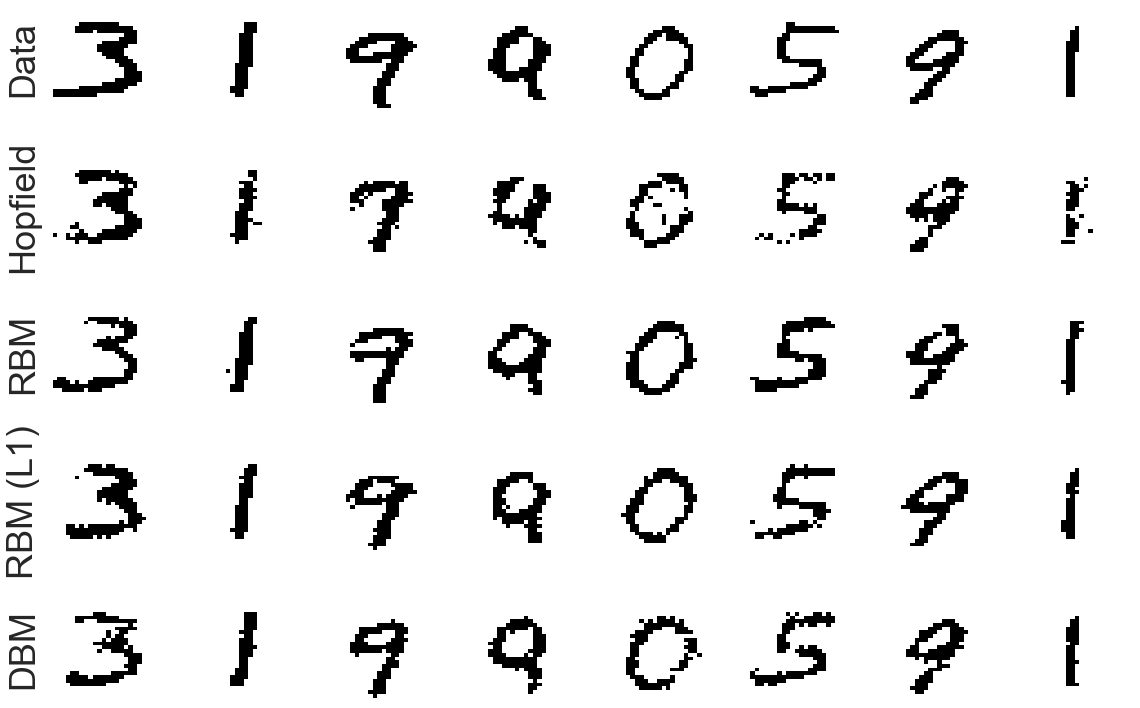### Fantasy Particles¶

Once we have the trained models ready, we can use MC to draw samples from the corresponding probability distributions, called "fantasy particles". To this end let us draw a random_sample from the validation data, and compute the model_state. Next, we define a MC sampler based on the model, and set its state to model_state. To compute the fantasy particles, we do layer-wise Gibbs sampling for a total of n_steps equilibration steps. The last step (controlled by the boolean mean_field) is a final mean-field iteration.

In :
def compute_fantasy_particles(model,num_fantasy,num_steps,mean_field=True):
"""
Draws samples from the model using Gibbs sampling Markov Chain Monte Carlo .
Starts from randomly initialized points.

Args:
model: a model
data: a tensor of shape (num_samples, num_visible_units)
num_steps (int): the number of update steps
mean_field (bool; optional): run a final mean field step to compute probabilities

Returns:
tensor of shape (num_samples, num_visible_units)

"""
schedule = schedules.Linear(initial=1.0, delta = 1 / (num_steps-1))
fantasy = samplers.SequentialMC.generate_fantasy_state(model,
num_fantasy,
num_steps,
schedule=schedule,
beta_std=0.0,
beta_momentum=0.0)
if mean_field:
fantasy = model.mean_field_iteration(1, fantasy)
fantasy_particles = fantasy.get_visible()
return be.to_numpy_array(fantasy_particles)

examples = data.get(mode='validate') # shape (batch_size, 784)
data.reset_generator(mode='validate') # reset the generator to the beginning of the validation set

hopfield_fantasy = compute_fantasy_particles(hopfield, num_to_plot, 100, mean_field=False)
rbm_fantasy = compute_fantasy_particles(rbm, num_to_plot, 100, mean_field=False)
rbm_L1_fantasy = compute_fantasy_particles(rbm_L1, num_to_plot, 100, mean_field=False)
dbm_fantasy = compute_fantasy_particles(dbm, num_to_plot, 100, mean_field=False)

fantasy_plot = plot_image_grid(
np.array([hopfield_fantasy,
rbm_fantasy,
rbm_L1_fantasy,
dbm_fantasy]),
image_shape, vmin=0, vmax=1, row_titles=["Hopfield", "RBM", "RBM (L1)", "DBM"])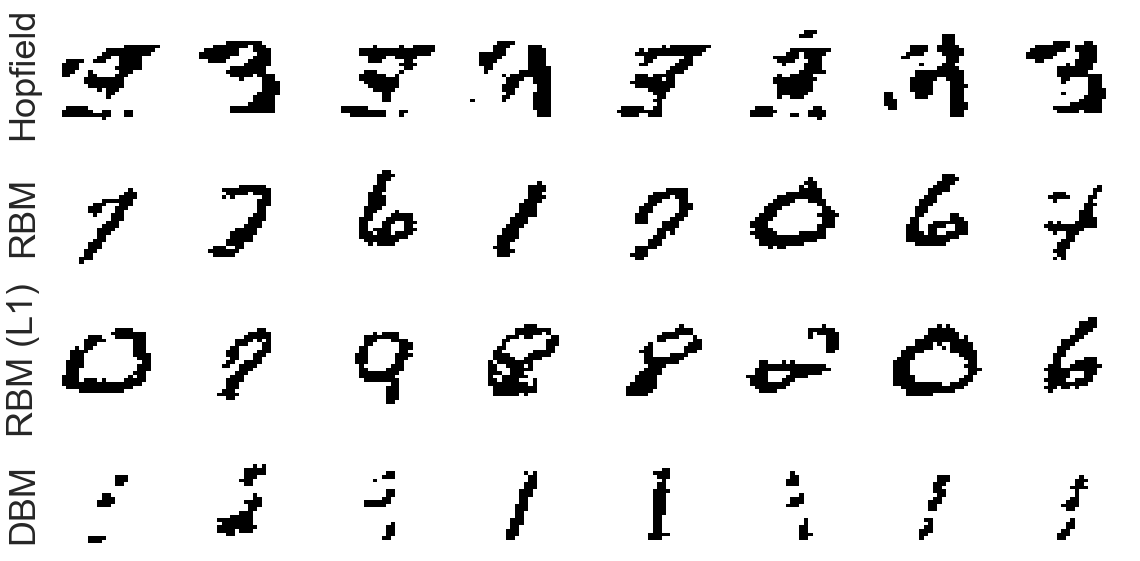### De-noising Images¶

One can use generative models to reduce the noise in images (de-noising). Let us randomly flip a fraction, fraction_to_flip, of the black & white bits in the validation data, and use the models defined above to reconstruct (de-noise) the digit images:

In :
##### denoise MNIST images
# get validation data
examples = data.get(mode='validate') # shape (batch_size, 784)
# reset data generator to beginning of the validation set
data.reset_generator(mode='validate')

# add some noise to the examples by randomly flipping some pixels 0 -> 1 and 1 -> 0
fraction_to_flip=0.15
# compute noisy data

# define number of digits to display
num_to_display=8
# compute de-noised images
hopfield_denoised=compute_reconstructions(hopfield,noisy_data[:num_to_display])
rbm_denoised=compute_reconstructions(rbm,noisy_data[:num_to_display])
rbm_L1_denoised=compute_reconstructions(rbm_L1,noisy_data[:num_to_display])
dbm_denoised=compute_reconstructions(dbm,noisy_data[:num_to_display])

denoising_plot = plot_image_grid(
np.array([examples[:num_to_plot],
noisy_data[:num_to_plot],
hopfield_denoised,
rbm_denoised,
rbm_L1_denoised,
dbm_denoised]),
image_shape, vmin=0, vmax=1, row_titles=["Data", "Noisy", "Hopfield", "RBM", "RBM (L1)", "DBM"])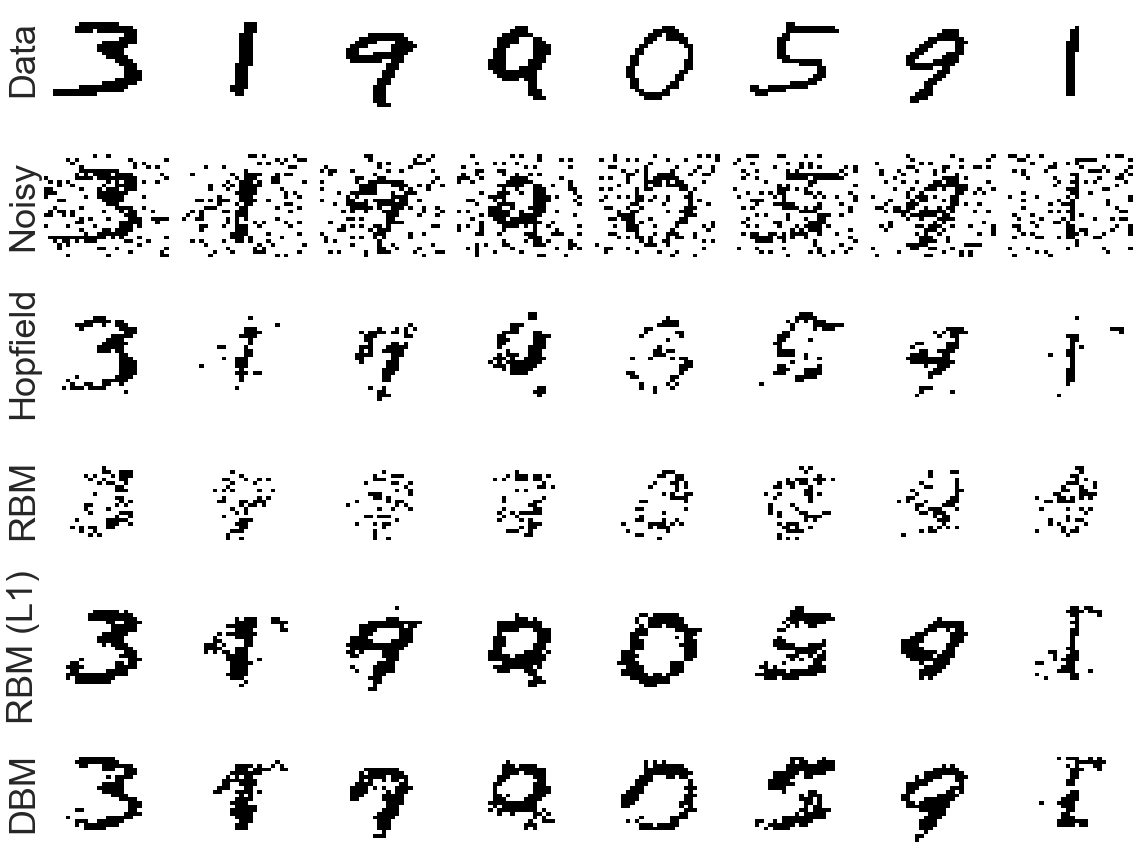### Weight Visualization¶

Let us open up the black box of our generative models now. Below, we show the features learned by the weights of the different models.

In :
# plot the weights of the hopfield model
hopfield_weights = plot_image_grid(
be.reshape(hopfield.connections.weights.W(trans=True)[:25], (5,5,784)),
image_shape,
vmin=be.tmin(hopfield.connections.weights.W()),
vmax=be.tmax(hopfield.connections.weights.W()),
cmap=cm.seismic)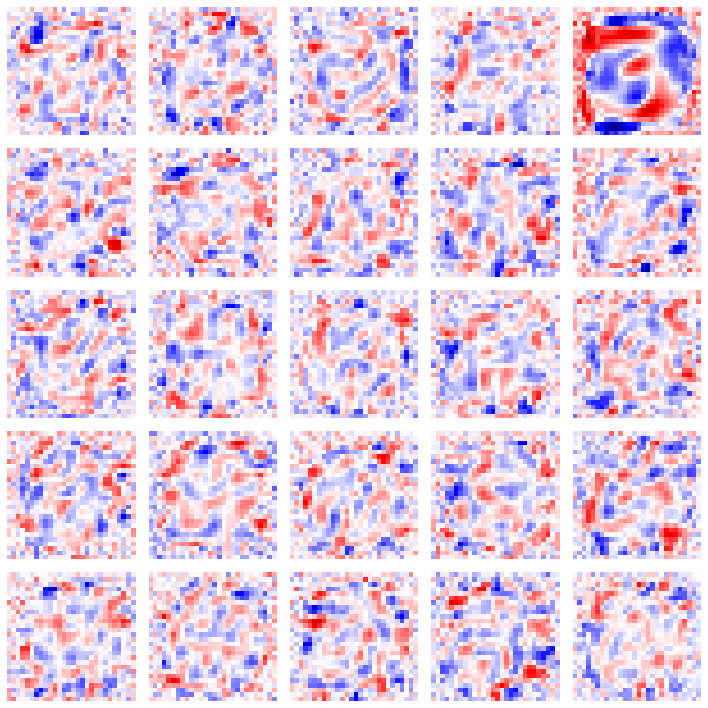In :
# plot the weights of the RBM
rbm_weights = plot_image_grid(
be.reshape(rbm.connections.weights.W(trans=True)[:25], (5,5,784)),
image_shape,
vmin=be.tmin(rbm.connections.weights.W()),
vmax=be.tmax(rbm.connections.weights.W()),
cmap=cm.seismic)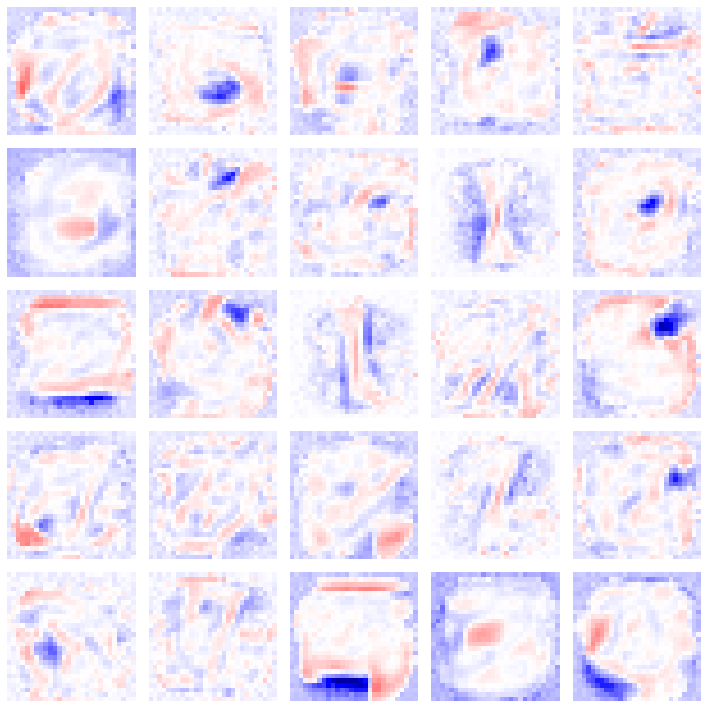In :
# plot the weights of the L1 regularized RBM
rbmL1_weights = plot_image_grid(
be.reshape(rbm_L1.connections.weights.W(trans=True)[:25], (5,5,784)),
image_shape,
vmin=be.tmin(rbm_L1.connections.weights.W()),
vmax=be.tmax(rbm_L1.connections.weights.W()),
cmap=cm.seismic)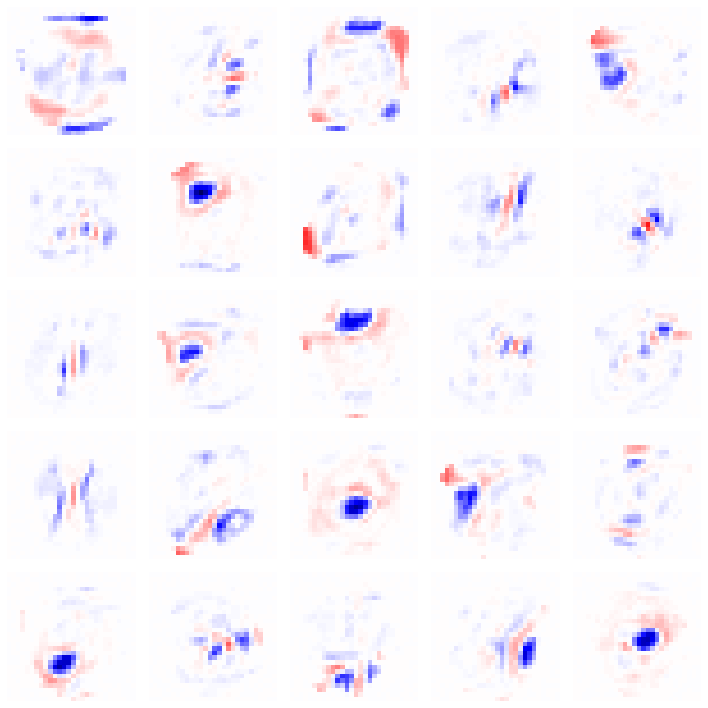In :
# plot the weights of the first layer of the dbm
dbm_weights = plot_image_grid(
be.reshape(dbm.connections.weights.W(trans=True)[:25], (5,5,784)),
image_shape,
vmin=be.tmin(dbm.connections.weights.W()),
vmax=be.tmax(dbm.connections.weights.W()),
cmap=cm.seismic)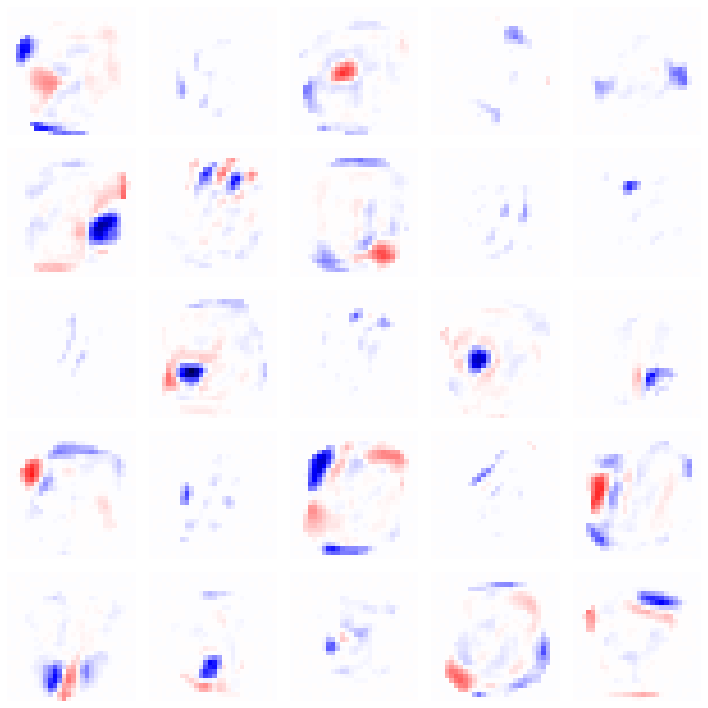In :
data.close() # close the HDF5 store with the MNIST dataset


## Exercises¶

• Try increasing/decreasing the number of hidden units and study systematically how the performance of the different models changes.
• Look up Paysage's documentation and study the performance for various SGD optimizers.